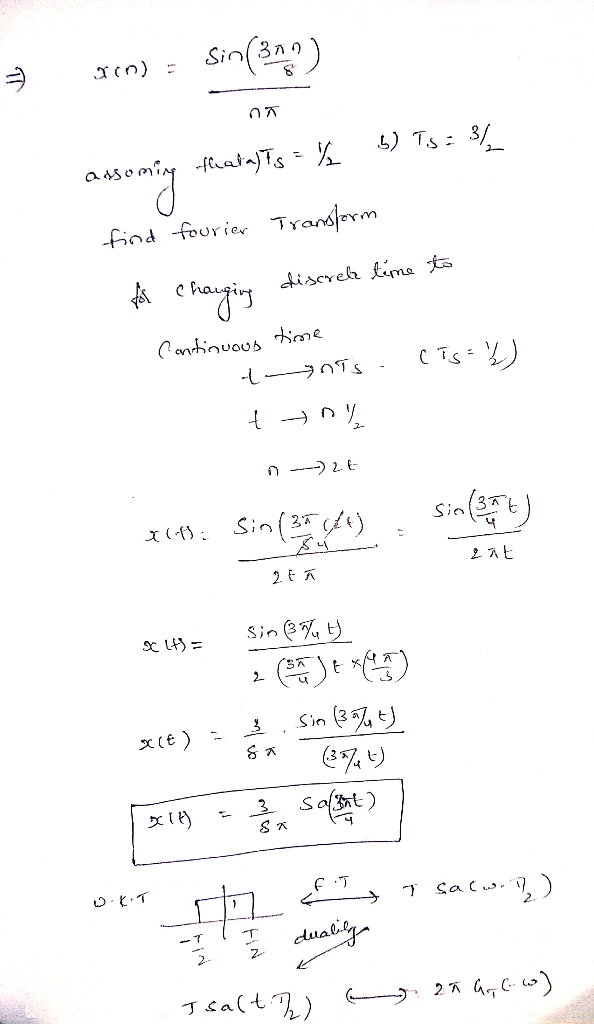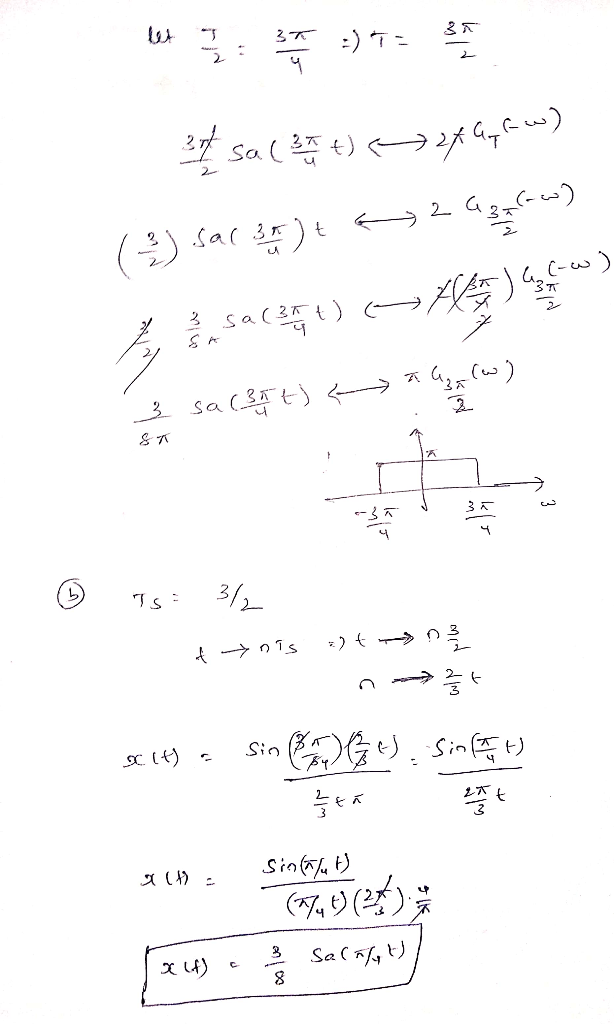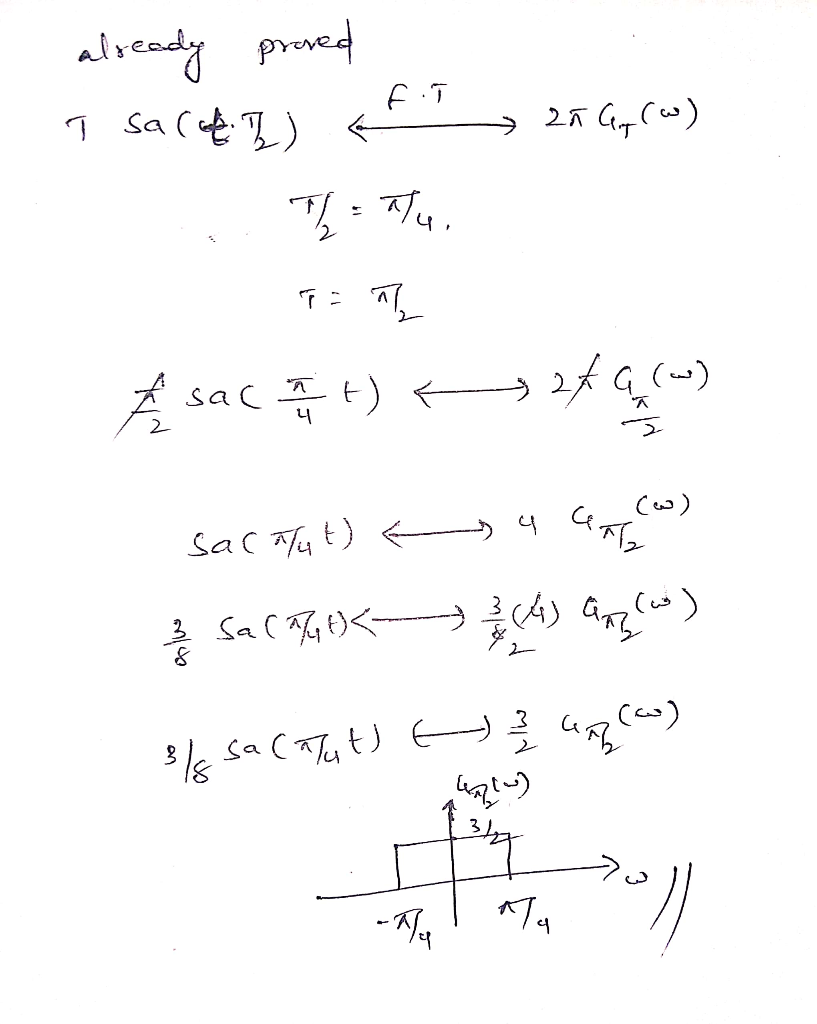Homework Help Question & Answers

Problem 4.8 Sketch the FT representation X6(ja) of the discrete-time signal x(n) = sin(3mm/8) ass...Problem 4.8 Sketch the FT representation X6(ja) of the discrete-time signal x(n) = sin(3mm/8) assuming that (a) T- 1/2, (b) T,-3/2. See Fia 4 19Add Answer of: Problem 4.8 Sketch the FT representation X6(ja) of the discrete-time signal x(n) = sin(3mm/8) ass...
More Homework Help Questions Additional questions in this topic.

• The only ideas that can be used include: area ABCI-RA2(A+B+C-lpi), the Pythagorean theorem: Cos c cos a cos b. Vectors-dot product cross product, sin A-sin a/sin c; cos A-cos a sin b/sin c; spher...

Need Online Homework Help?

Get FREE EXPERT Answers
WITHIN MINUTES
Related Questions